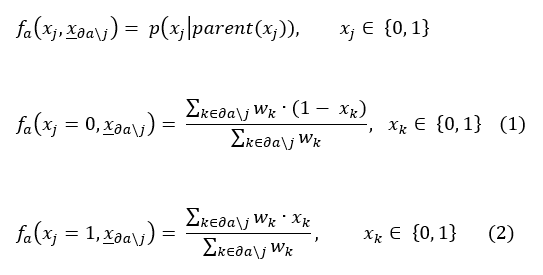# 1.0 - 8.00 - LoopyBeliefPropagation Background - Teradata Vantage

## Teradata® Vantage Machine Learning Engine Analytic Function Reference

Product
Release Number
1.0
8.00
Release Date
May 2019
Content Type
Programming Reference
Publication ID
B700-4003-098K
Language
English (United States)

A Bayesian network represents a set of random variables and their conditional dependencies. For example, a Bayesian network can represent the probabilistic relationships between symptoms and diseases. Given symptoms, belief propagation can use the graph to compute the probabilities of the presence of various diseases.

Formally, a Bayesian network is a directed acyclic graph (DAG) whose vertices (or nodes) represent random variables in the Bayesian sense: They are observable quantities, latent variables, unknown parameters, or hypotheses. Each vertex is associated with a probability function that takes the values of the vertex parent variables and returns the probability of the variable represented by the vertex. For example, if the parents are m binary variables, the probability function is represented by a table of 2 m entries, one entry for each of the 2 m possible combinations of its parent values. If variables are conditionally dependent on each other, the vertices that represent them are connected by edges.

For example, suppose that there are two reasons that grass can be wet: the sprinkler is on or it is raining. Also, suppose that when it is raining, the sprinkler is less likely to be on. The Bayesian network in the following figure models this situation. The three variables are binary; their possible values are T (true) and F (false).To use the LoopyBeliefPropagation function, you must specify only the conditional dependence between variables (directed edges, possibly weighted) and the values for observed variables. The function computes the potential tables, using these functions at factor nodes: